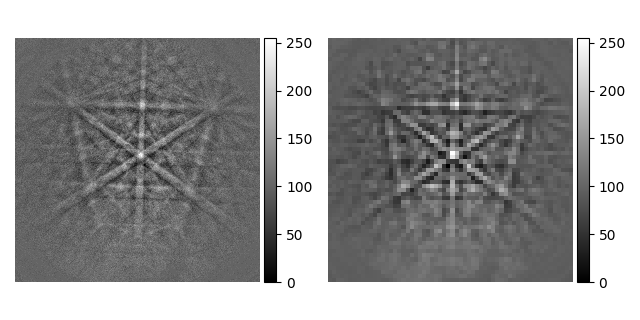# Pattern binning#

This example shows how to bin `EBSD` patterns using `downsample()` or HyperSpy’s `rebin()` (see Rebinning for details).

Note

In general, better contrast is obtained by removing the static (and dynamic) background prior to binning instead of after it.

```import hyperspy.api as hs
import kikuchipy as kp

s.remove_static_background(show_progressbar=False)

print(s)
print(s.static_background.shape)
print(s.detector)
```
```<EBSD, title: si_in Scan 1, dimensions: (|480, 480)>
(480, 480)
EBSDDetector (480, 480), px_size 1.0 um, binning 1, tilt 0.0, azimuthal 0.0, pc (0.5, 0.5, 0.5)
```

Downsample by a factor of 8 while maintaining the data type (achieved by rescaling the pattern intensity). Note how the `static_background` and `detector` attributes are updated.

```s2 = s.downsample(8, inplace=False)
_ = hs.plot.plot_images([s, s2], axes_decor="off", tight_layout=True, label=None)
print(s2.static_background.shape)
print(s2.detector)
``````[                                        ] | 0% Completed | 265.08 us
[########################################] | 100% Completed | 100.68 ms
(60, 60)
EBSDDetector (60, 60), px_size 1.0 um, binning 8, tilt 0.0, azimuthal 0.0, pc (0.5, 0.5, 0.5)
```

Rebin by passing the new shape (use `(1, 1, 60, 60)` if binning a 2D map). Note how the pattern is not rescaled and the data type is cast to either `int64` or `float64` depending on the initial data type.

```s3 = s.rebin(new_shape=(60, 60))
print(s3.data.dtype)
print(s3.data.min(), s3.data.max())
```
```uint64
3152 11998
```

The latter method is more flexible in that it allows for different binning factors in each axis, the factors are not restricted to being integers and the factors do not have to be divisors of the initial signal shape.

```s4 = s.rebin(scale=(8, 9))
print(s4.data.shape)
```
```(53, 60)
```

Total running time of the script: (0 minutes 4.802 seconds)

Estimated memory usage: 35 MB

Gallery generated by Sphinx-Gallery